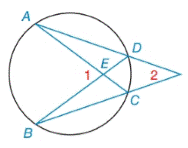Chapter 6.2, Problem 11EElementary Geometry For College St...

7th Edition
Alexander + 2 others
ISBN: 9781337614085

Solutions

Chapter
SectionElementary Geometry For College St...

7th Edition
Alexander + 2 others
ISBN: 9781337614085
Textbook Problem

Given: m ∠ 2 =36 ° m A B ⌢ = 4 ⋅ m D C ⌢ Find: a) m A B ⌢ b) m ∠ 1To determine

(a)

To find:

mAB when m2=36° and mAB=4mDC.

Explanation

Theorem:

The measure of an angle formed when two secants intersects at a point outside the circle is one-half the difference of the measures of the two intercepted arcs.

Calculation:

mAB when m2=36° and mAB=4mDC.

By Theorem,

Let, mDC=x.

m2=12(mABmDC)36°=12(4×xx)36°=

To determine

(b)

To find:

m1 when mAB=96° and mDC=24°.

Still sussing out bartleby?

Check out a sample textbook solution.

See a sample solution

The Solution to Your Study Problems

Bartleby provides explanations to thousands of textbook problems written by our experts, many with advanced degrees!

Get Started

Find more solutions based on key concepts